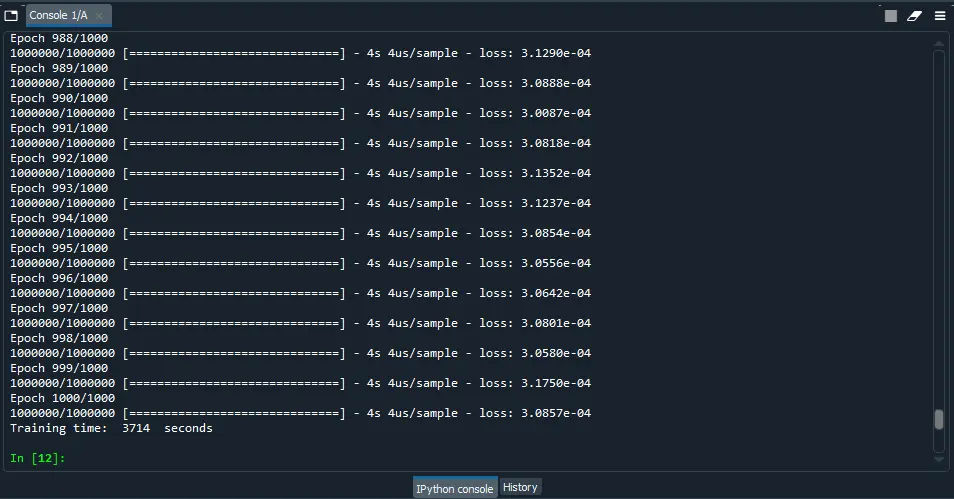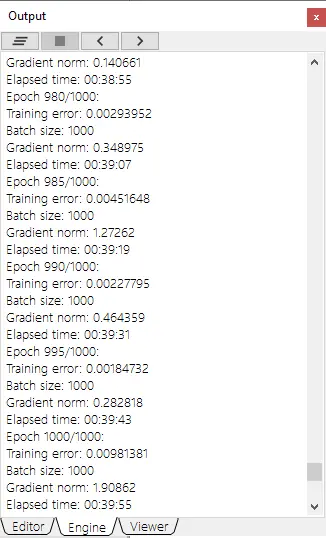﻿ Performance comparison of dense networks in GPU: TensorFlow vs PyTorch vs Neural Designer

# Performance comparison of dense networks in GPU to Neural Designer

By Carlos Barranquero, Artelnics. 1 December 2020.TensorFlow, PyTorch and Neural Designer are three popular machine learning platforms developed by Google, Facebook and Artelnics, respectively.

Although all that frameworks are based on neural networks, they present some important differences in functionality, usability, performance, etc.

This post compares the GPU training speed of TensorFlow, PyTorch and Neural Designer for an approximation benchmark.

As we will see, Neural Designer trains this neural network x1.55 times faster than TensorFlow and x2.50 times faster than PyTorch in a NVIDIA Tesla T4.

In this article, we provide all the steps that you need to reproduce the results using the free trial of Neural Designer.

Contents:

## Introduction

One of the most important factors in machine learning platforms is their training speed. Indeed, modelling huge data sets is very expensive in computational terms.Major machine learning tools use GPU computing techniques, such as NVIDIA CUDA, to speed up model training.

This article aims to measure the GPU training times of TensorFlow, PyTorch and Neural Designer for a benchmark application and compare the speeds obtained by those platforms.

The following table summarizes the technical features of these tools that might impact their GPU performance.

TensorFlow PyTorch Neural Designer
Written in C++, CUDA, Python C++, CUDA, Python C++, CUDA
Interface Python Python Graphical User Interface
Differentiation Automatic Automatic Analytical

The above table shows that TensorFlow and PyTorch are programmed in C++ and Python, while Neural Designer is entirely programmed in C++.

Interpreted languages like Python have some advantages over compiled languages like C ++, such as their ease of use.

However, the performance of Python is, in general, lower than that of C++. Indeed, Python takes significant time to interpret sentences during the program's execution.

On the other hand, TensorFlow and PyTorch use automatic differentiation, while Neural Designer uses analytical differentiation.

As before, automatic differentiation has some advantages over analytical differentiation. Indeed, it simplifies obtaining the gradient for new architectures or loss indices.

However, the performance of automatic differentiation is, in general, lower than that of analytical differentiation: The first derives the gradient during the program's execution, while the second has that formula pre-calculated.

Next, we measure the training speed for a benchmark problem on a reference computer using TensorFlow, PyTorch and Neural Designer. The results produced by that platforms are then compared.

## Benchmark application

The first step is to choose a benchmark application that is general enough to draw conclusions about the performance of the machine learning platforms. As previously stated, we will train a neural network that approximates a set of input-target samples.

In this regard, an approximation application is defined by a data set, a neural network and an associated training strategy. The next table uniquely defines these three components.

Data setBenchmark: Rosenbrock Inputs number: 1000 Targets number: 1 Samples number: 1000000 File size: 22 Gb (download) Layers number: 2 Layer 1: -Type: Perceptron (Dense) -Inputs number: 1000 -Neurons number: 1000 -Activation function: Hyperbolic tangent (tanh) Layer 2: -Type: Perceptron (Dense) -Inputs number: 1000 -Neurons number: 1 -Activation function: Linear Initialization: Random uniform [-1,1] Loss index: -Error: Mean Squared Error (MSE) -Regularization: None Optimization algorithm: -Algorithm: Adaptive Moment Estimation (Adam) -Batch size: 1000 -Maximum epochs: 1000

Once the TensorFlow, PyTorch and Neural Designer applications have been created, we need to run them.

## Reference computer

The next step is to choose the computer to train the neural networks with TensorFlow, PyTorch and Neural Designer. For training speed tests, the most important feature of the computer is the GPU or device card.

All calculations have been done on an Amazon Web Services instance to make the results easier to reproduce. The following table lists some basic information about the computer used here.

Instance type: AWS g4dn.xlarge Windows 10 Enterprise CPU Intel(R) Xeon(R) Platinum 8259CL CPU @ 2.50GHz 16.0 GB NVIDIA Tesla T4

Once the computer has been chosen, we install TensorFlow (2.1.0), PyTorch (1.7.0) and Neural Designer (5.0.4) on it.

#TENSORFLOW CODE
import tensorflow as tf
import pandas as pd
import time
import numpy as np

start_time = time.time()
filename = "C:/R_new.csv"
df_test = pd.read_csv(filename, nrows=100)
float_cols = [c for c in df_test if df_test[c].dtype == "float64"]
float32_cols = {c: np.float32 for c in float_cols}
data = pd.read_csv(filename, engine='c', dtype=float32_cols)
print("Loading time: ", round(time.time() - start_time), " seconds")

x = data.iloc[:,:-1].values
y = data.iloc[:,[-1]].values

initializer = tf.keras.initializers.RandomUniform(minval=-1., maxval=1.)

#build model
model = tf.keras.models.Sequential([tf.keras.layers.Dense(1000,
activation = 'tanh',
kernel_initializer = initializer,
bias_initializer=initializer),
tf.keras.layers.Dense(1,
activation = 'linear',
kernel_initializer = initializer,
bias_initializer=initializer)])

#compile model
model.compile(optimizer='adam', loss = 'mean_squared_error')

#train model
start_time = time.time()
history = model.fit(x, y, batch_size = 1000, epochs = 1000)
print("Training time: ", round(time.time() - start_time), " seconds")


Building this application with PyTorch also requires some Python scripting. This code is listed below. Also, you can download here.

#PYTORCH CODE
import pandas as pd
import time
import torch
import numpy as np
import statistics

def init_weights(m):
if type(m) == torch.nn.Linear:
torch.nn.init.uniform_(m.weight, a=-1.0, b=1.0)
torch.nn.init.uniform_(m.bias.data, a=-1.0, b=1.0)

epoch = 1000
total_samples, batch_size, input_variables, hidden_neurons, output_variables = 1000000, 1000, 1000, 1000, 1
device = torch.device("cuda:0")

# read data float32
start_time = time.time()
filename = "C:/R_new.csv"
df_test = pd.read_csv(filename, nrows=100)
float_cols = [c for c in df_test if df_test[c].dtype == "float64"]
float32_cols = {c: np.float32 for c in float_cols}
dataset = pd.read_csv(filename, engine='c', dtype=float32_cols)
print("Loading time: ", round(time.time() - start_time), " seconds")

x = torch.tensor(dataset.iloc[:,:-1].values, dtype = torch.float32)
y = torch.tensor(dataset.iloc[:,[-1]].values, dtype = torch.float32)
# build model
model = torch.nn.Sequential(torch.nn.Linear(input_variables, hidden_neurons),
torch.nn.Tanh(),
torch.nn.Linear(hidden_neurons, output_variables)).cuda()

# initialize weights
model.apply(init_weights)

# compile model
learning_rate = 0.001
loss_fn = torch.nn.MSELoss(reduction = 'mean')
optimizer = torch.optim.Adam(model.parameters(), lr=learning_rate)

indices = np.arange(0,total_samples)

start = time.time()

for j in range(epoch):

mse=[]

t0 = time.time()

for i in range(0, total_samples, batch_size):

batch_indices = indices[i:i+batch_size]

batch_x, batch_y = x[batch_indices], y[batch_indices]

batch_x = batch_x.cuda()

batch_y = batch_y.cuda()

outputs = model.forward(batch_x)

loss = loss_fn(outputs, batch_y)

loss.backward()

optimizer.step()

mse.append(loss.item())

print("Epoch:", j+1,"/1000", "[================================] - ","loss: ", statistics.mean(mse))

t1 = time.time() - t0

print("Elapsed time: ", int(round(t1 )), "sec")

end = time.time()

elapsed = end - start

print("Training time: ",int(round(elapsed )), "seconds")


Once the TensorFlow, PyTorch and Neural Designer applications have been created, we need to run them.

## Results

The last step is to run the benchmark application on the selected machine with TensorFlow, PyTorch and Neural Designer and to compare the training times provided by those platforms.

The next figure shows the training results with TensorFlow.As we can see, TensorFlow takes 3,714 seconds (01:01:54) to train the neural network for 1000 epochs. The final mean squared error is 0.0003. With TensorFlow, the average GPU usage during training is 45% approximately.

Similarly, the following figure is a screenshot of PyTorch at the end of the process.In this case, PyTorch takes 6,006 seconds (01:40:06) to train the neural network for 1000 epochs, reaching a mean squared error of 0.00593. With PyTorch, the average GPU usage during training is 40% approximately.

Finally, the following figure shows the training results with Neural Designer.Neural Designer takes 2,395 seconds (00:39:55) to train the neural network for 1000 epochs. During that time, it reaches a mean squared error of 0.00981. With Neural Designer, the average GPU usage during training is 95% approximately.

The following table summarizes the the most important metrics that the three machine learning platforms yielded .

TensorFlow PyTorch Neural Designer
Training time 01:01:54 01:40:06 00:39:55
Epoch time 3.714 seconds/epoch 6.006 seconds/epoch 2.395 seconds/epoch
Training speed 269,251 samples/second 166,500 samples/second 417,537 samples/second

Finally, the following chart depicts the training speeds of TensorFlow, PyTorch and Neural Designer graphically for this case.As we can see, the training speed of Neural Designer for this application is x1.55 times bigger than that of TensorFlow and x2.50 times bigger than that of PyTorch.

## Conclusions

Neural Designer is entirely written in C ++, uses analytical differentiation, and has been optimized to minimize the number of operations during training.

This means that, for the benchmark described in this post, its training speed is x1.55 times faster than that of TensorFlow and x2.50 times faster than that of PyTorch.

To reproduce these results, download the free trial of Neural Designer and follow the steps described in this article.